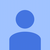0

# diagonalize symmetric matrices

Junk Smith 5 years ago updated by Pavel Holoborodko 4 years ago
Hi,
Is there any method that provides a speed up (over eig) for symmetric or Hermitian matrices? What is your current time estimate for diagonalizing a 1000x1000 real, symmetric matrix for quad precision?

Finally, how does the time to diagonalize scale with matrix size D at a fixed precision? Is it still D^3 as for double precision?Under review
Hi,

1. Of course, the "eig" routine in toolbox uses different algorithms depending on matrix properties.
In particular, it uses MRRR in symmetric/Hermitian case. It is current state of the art delivering near O(n^2) complexity.
(see references on the page: http://goo.gl/L2Nzrv and also this link: http://goo.gl/v9iSxq).

2. Time estimate depends on CPU/number of cores, etc. On my computer with Core i7 (4 HW cores) I see following results for quadruple precision:
```>> A = mp(rand(1000)-0.5);
>> A = A+A';
>> tic; eig(A); toc;
Elapsed time is 32.662471 seconds.
```
Unsymmetric case for comparison:
```>> A = mp(rand(1000)-0.5);
>> tic; eig(A); toc;
Elapsed time is 134.259668 seconds.
```

3. MATLAB/VPA, Maple and Mathematica need hours (days in case of VPA) to handle these problems, as all are using the standard QR iteration which is O(n^3).

4. Our trial version is freely accessible from our website - you can test the toolbox in your environment in a minutes.

Let me know if you have any other questions.
Pavel.Also in case of symmetric/Hermitian matrix you can choose what method to use, by using the second argument to "eig":
```>> A = mp(rand(1000)-0.5);
>> A = A+A';
>> tic; eig(A); toc;          % use default, which is MRRR
Elapsed time is 32.662471 seconds.

>> tic; eig(A,'dc'); toc;     % use divide & conquer
Elapsed time is 31.053027 seconds.

>> tic; eig(A,'mr'); toc;     % use MRRR
Elapsed time is 32.703078 seconds.
```
MRRR and divide & conquer are both optimized for symmetric matrices. Generally timings are comparable, but we use MRRR by default as it shows better results over wide range of matrices.

Second parameter of "eig" is toolbox-specific, don't try using it with built-in routine :).Hi Pavel,
Thanks for your reply. Can you point me on instructions for how to download the trial version for mac OS X? The right panel on http://www.advanpix.com/ only opened a windows application.Hi again,

Thank you,
Pavel.Your question made me to revise the parallel optimization of eigensolver for symmetric/Hermitian matrices.

As a result, today we have released new version of toolbox which solves the symmetric eigenproblem by ~3.4 times faster.
So that eig(A) for symmetric 1Kx1K matrix now takes around 9 seconds on the same CPU.

Larger matrices receive higher speed-up ratio. Actually now solver scales almost linearly to number of cores.For future references, here is architecture of eigensolver implemented in toolbox:

In some cases we are faster than even double precision EIG of MATLAB.